Next: Terminology Up: Subset-Sum Problems and NP-Completeness Previous: Converting (SSP) to (SP)   Contents

## Cook's Theorem

It is more important to understand the general idea of what we did in section 5.3 than to get involved in the details of the construction of the adding machine.'' We used logical variables (the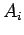) to represent our guess as to what a solution to the (SSP) was, then constructed a set of formulas to verify the guess was correct.

You should be able to convince yourself that a similar thing could be done with a factoring problem. We could have variablesand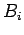represent our guesses as to two factors, then construct a multiplication machine'' to verify that the product is what we want. Thus an efficient algorithm for (SP) would lead to an efficient algorithm for factoring6.

Many other problems can be viewed as making some guesses as to what the correct answer is, with the process of verification relatively easy. An example might be an integer programming problem: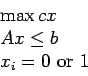We ask if there is a feasible solution with objective function value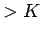. For a given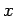, it is easy to check that it satisfies all the problem constraints and tell if the value is big enough.

The detailed construction in section 5.3 was intended primarily to convince you that, if a verification can be done efficiently, it can be simulated by a set of logic formulas. It should make you willing to believe

Theorem 18 (Cook)   Any guess and verify'' problem can be converted to a satisfiability problem. Thus, an efficient algorithm for (SP) can efficiently solve any guess and verify'' problem.

#### Footnotes

... factoring6
Unlike (SSP), nobody has been able to show that an algorithm for factoring would give an algorithm for (SP).Next: Terminology Up: Subset-Sum Problems and NP-Completeness Previous: Converting (SSP) to (SP)   Contents
Translated from LaTeX by Scott Sutherland
2002-12-14